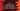# C++ program to capitalize first and last character of each word in a string## C++ program to capitalize first and last character of each word in a string:

One string is given. We need to capitalize first and last character of each word in this string in C++. C++ provides toupper method that can be used to convert one character to uppercase or capitalize.

We will write one for loop to iterate through the characters of the string one by one. Inside this loop, we will capitalize the character if it is:

• First or last character
• If there is a space, capitalize the character before and after it. This step is used to handle all middle words in the string.

### C++ program:

Let’s write down the program:

``````#include <iostream>
using namespace std;

string capitalizeCharStr(string givenString)
{
for (int i = 0; i < givenString.length(); i++)
{
if (i == 0 || i == givenString.length() - 1)
{
givenString[i] = toupper(givenString[i]);
}
else if (givenString[i] == ' ')
{
givenString[i - 1] = toupper(givenString[i - 1]);
givenString[i + 1] = toupper(givenString[i + 1]);
}
}
return givenString;
}

int main()
{
string givenStr = "hello world and Hello universe ";
cout << "Given string : " << givenStr << endl;
cout << capitalizeCharStr(givenStr) << endl;
}``````

### Explanation of this program:

1. capitalizeCharStr method is used to capitalize the first and last character of each word in the string. It takes one string and returns the modified string.
2. We are using one for loop to iterate through the characters of the string one by one.
3. We have one if condition and one else if condition. The if condition checks if the current character is first or last. It capitalizes the character if this condition is true. The else if block checks if the current character is a blank space. If it is a blank space, it indicates that it is the space between two words. In that case, we are capitalizing the character just before and after this blank space.

### Output:

If you run the above program, it will print the below output:

``````Given string : hello world and Hello universe
HellO WorlD AnD HellO Universe ``````

You can try to run the program with different types of strings.